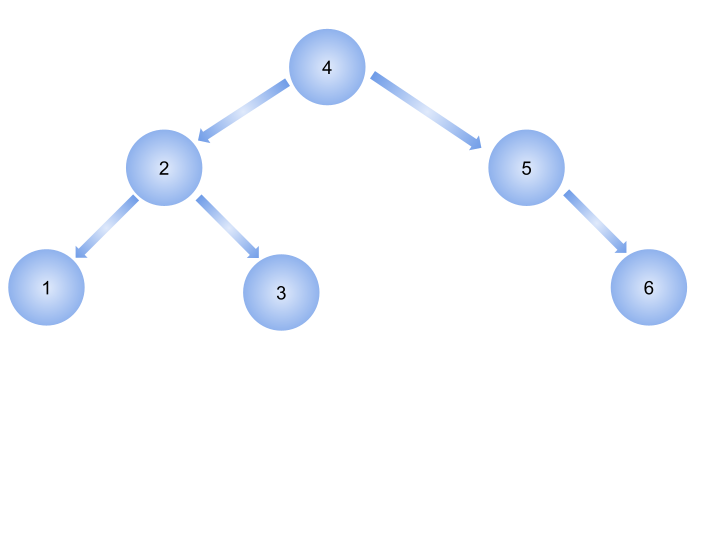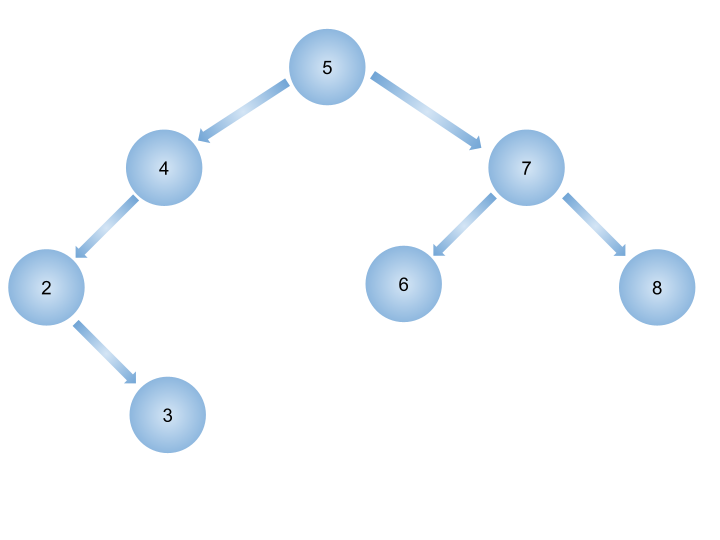# BST Sequences

Posted: 26 Feb, 2021
Difficulty: Hard

## PROBLEM STATEMENT

#### You are given a binary search tree consisting of distinct elements. The binary search tree is created by traversing through the sequence from left to right and inserting each element. You need to print all the sequences or ways that would result in creating the given BST.

##### Note:
``````Keep in mind that while merging the sequences the relative order of elements should be preserved.
``````
##### For example :
``````For the given binary search tree
````````````The valid BST sequences for the above BST are:
4 2 1 3 5 6
4 2 1 5 3 6
4 2 1 5 6 3
4 2 3 1 5 6
4 2 3 5 1 6
4 2 3 5 6 1
4 2 5 1 3 6
4 2 5 1 6 3
4 2 5 3 1 6
4 2 5 3 6 1
4 2 5 6 1 3
4 2 5 6 3 1
4 5 2 1 3 6
4 5 2 1 6 3
4 5 2 3 1 6
4 5 2 3 6 1
4 5 2 6 1 3
4 5 2 6 3 1
4 5 6 2 1 3
4 5 6 2 3 1

You need to print all of them.
``````
##### Input Format:
``````The first line contains an integer 'T' which denotes the number of test cases or queries to be run. Then the test cases are as follows.

The first line of each test case contains elements of the tree in the level order form. The line consists of values of nodes separated by a single space. In case a node is null, we take -1 in its place.

For example, the input for the tree depicted in the below image would be :
````````````Input Format:
5
4 7
2 -1 6 8
-1 3 -1 -1 -1 -1
-1 -1

Explanation :
Level 1 :
The root node of the tree is 5

Level 2 :
Left child of 5 = 4
Right child of 5 = 7

Level 3 :
Left child of 4 = 2
Right child of 4 = null (-1)
Left child of 7 = 6
Right child of 7 = 8

Level 4 :
Left child of 2 = null (-1)
Right child of 2 = 3
Left child of 6 = null (-1)
Right child of 6 = null (-1)
Left child of 8 = null (-1)
Right child of 8 = null (-1)

Level 5 :
Left child of 3 = null (-1)
Right child of 3 = null (-1)

The first not-null node(of the previous level) is treated as the parent of the first two nodes of the current level. The second not-null node (of the previous level) is treated as the parent node for the next two nodes of the current level and so on.
The input ends when all nodes at the last level are null(-1).
``````
##### Note :
``````The above format was just to provide clarity on how the input is formed for a given tree.
The sequence will be put together in a single line separated by a single space. Hence, for the above-depicted tree, the input will be given as:

5 4 7 2 -1 6 8 -1 3 -1 -1 -1 -1 -1 -1
``````
##### Output Format:
``````For each test case, print all the valid BST sequences of the given Binary Search Tree in a separate line.

Print the output of each test case in sorted order.
Print the output of each test case in a separate line.
``````
##### Note :
``````You do not need to print anything; it has already been taken care of. You just need to store all valid sequences of the given BST in a predefined data structure.
``````
##### Constraints:
``````1 <= T <= 10
0 <= N <= 10
1 <= data <= 10^4

Time Limit: 1sec
``````Approach 1

The basic idea of solving this question is to use the divide and conquer approach. The main idea behind this approach of generating sequences is that the “root” node is always going to be the first element of all possible sequences.

So, we will always start with the “root” node of the tree, as it is the only valid choice. Now for each of the rest valid choices, we will:

• Remove one of the valid choices and add its child nodes to the set of choices.
• Now, recursively find all the possible solutions for the new set of choices.
• Finally, append the root to the head of each of these solutions.

The recursion will end when we don't have any remaining nodes or choices left.

• If the “node” is NULL.
• If the “node” is the last child node.

After creating the base cases we will divide the tree into left and right subtrees and recursively call for both these trees, and finally merging the solutions from the two subtrees.

Algorithm:

• Create the first base case:
• If “node” is NULL, then simply return an empty sequence:
• Create the second base case:
• If “node” is the last child node, then return the value of that node.
• Now, for the solution we will recursively call for the left and the right subtrees and get a separate subsequence from each of the subtrees, let's say “leftSeq” and “rightSeq”.
• Now, we have two subsequences and we just need to merge them into a single sequence. The problem is that you should be careful with the relative order of the merged sequence.
• To do this, we create a bool vector, let’s say “flag” and fill it with “left” 0's and “right” 1's, where “left” is the size of the left sequence and “right” is the size of the right sequence.
• A value of 0 represents a member from the left sequence and a value of 1 represents a member from the right sequence.
• Now we generate all permutations of this “flag” vector, for which we use the “next_permutation” method.
• Now for each permutation of the “flag” vector, we will have a distinct merged sequence of “leftSeq” and “rightSeq”.
• Finally add the value of the current node at the start of each merged sequence, because the root must come before all child nodes.
• Return the sequence.